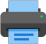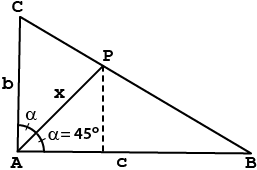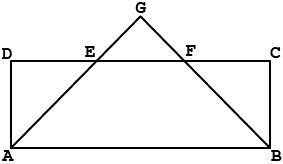Lista de exercícios do ensino médio para impressãoSelect all.
print selected exercises
Knowing that in the right triangle ABC shown in the picture b = 1 and c = 2, find x.
a)
$\,\sqrt{2}\,$
b)
$\,\dfrac{3}{2}\,$
c)
$\,\dfrac{3\sqrt{2}}{2}\,$
d)
$\,\dfrac{2}{3}\,$
e)
$\,\dfrac{2\sqrt{2}}{3}\,$×
The length of the two parallel sides of a right trapezoid are 6 cm and 8 cm, respectively top and bottom bases, and the altitude is 4 cm. The distance between the intersection of the two straight lines that contain the non-parallel legs and the midpoint of the major base is:
a)
$\,5\sqrt{15}\,$ cm
b)
$\,2\sqrt{19}\,$ cm
c)
$\,3\sqrt{21}\,$ cm
d)
$\,4\sqrt{17}\,$ cm
e)
none of these

×
In the picture below, ABCD is a rectangle, $\,\overline{AB}\,=\,4\,$, $\,\overline{BC}\,=\,1\;$ and $\,\overline{DE}\,=\,\overline{EF}\,=\,\overline{FC}\;$. The length of $\,\overline{BG}\,$ is:
a)
$\,\dfrac{\sqrt{5}}{4}\,$
b)
$\,\dfrac{5}{2}\,$
c)
$\,\dfrac{9}{4}\,$
d)
$\,\dfrac{11}{4}\,$
e)
$\,\dfrac{5}{\sqrt{2}}\,$